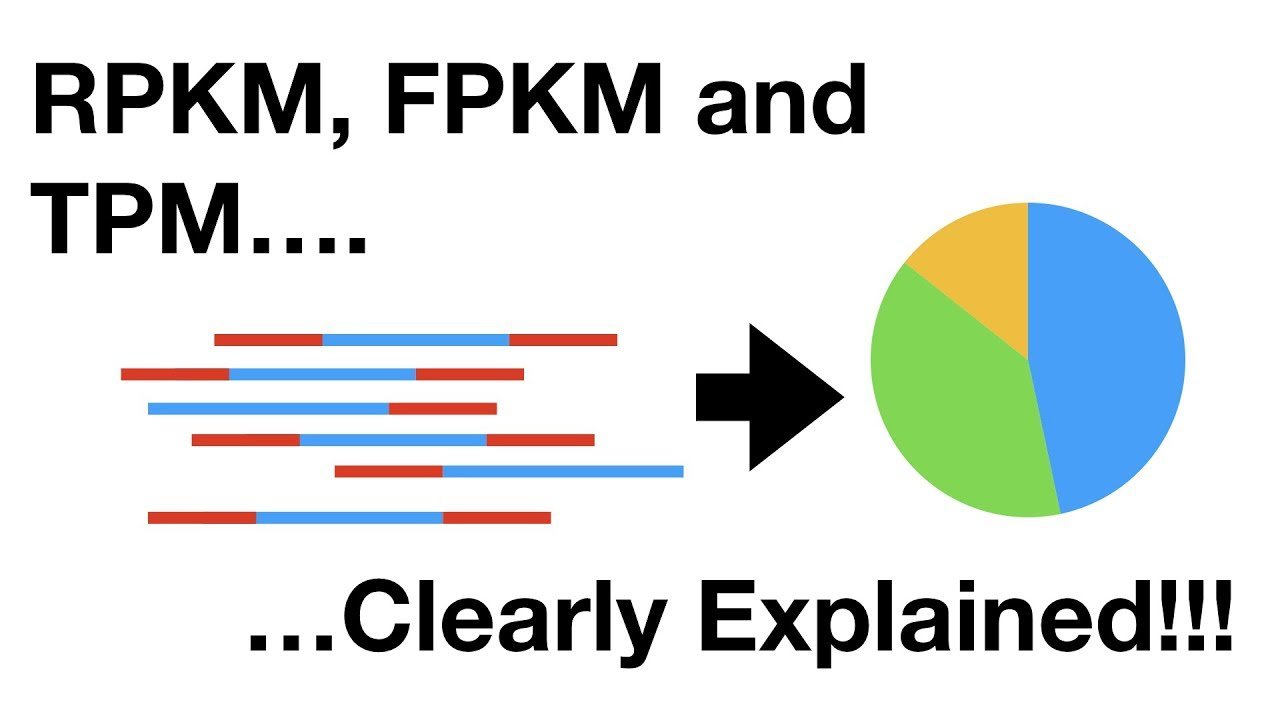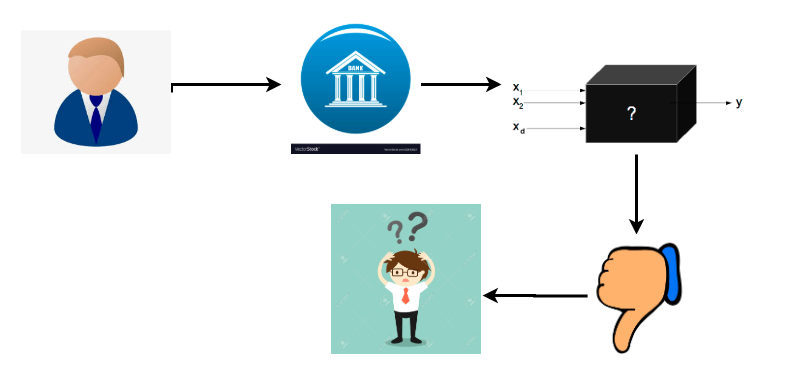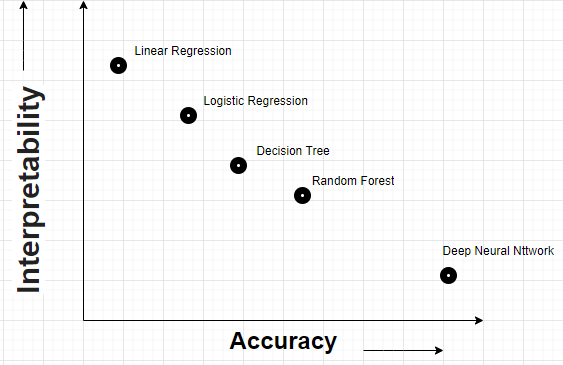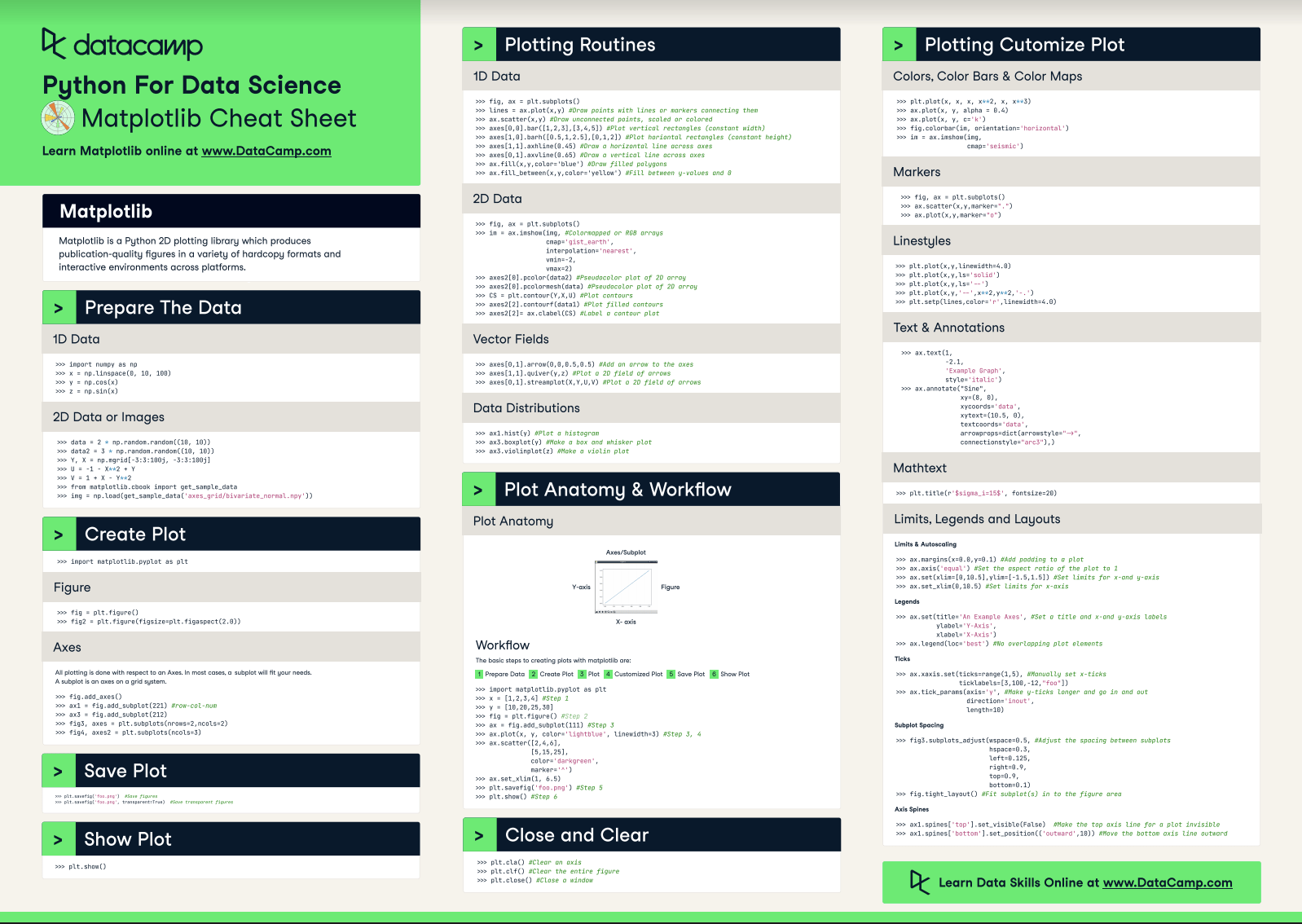1624031160

# RPKM, FPKM and TPM, Clearly Explained!!!

A StatQuest about RPKM, FPKM and TPM. These terms are for high-throughput RNA-seq experiments.

http://statquest.org/

#machine-learning

## Buddha Community1624031160

## RPKM, FPKM and TPM, Clearly Explained!!!

A StatQuest about RPKM, FPKM and TPM. These terms are for high-throughput RNA-seq experiments.

http://statquest.org/

#machine-learning1604178900

## Explaining the Explainable AI: A 2-Stage Approach

As artificial intelligence (AI) models, especially those using deep learning, have gained prominence over the last eight or so years , they are now significantly impacting society, ranging from loan decisions to self-driving cars. Inherently though, a majority of these models are opaque, and hence following their recommendations blindly in human critical applications can raise issues such as fairness, safety, reliability, along with many others. This has led to the emergence of a subfield in AI called explainable AI (XAI) . XAI is primarily concerned with understanding or interpreting the decisions made by these opaque or black-box models so that one can appropriate trust, and in some cases, have even better performance through human-machine collaboration .

While there are multiple views on what XAI is  and how explainability can be formalized [4, 6], it is still unclear as to what XAI truly is and why it is hard to formalize mathematically. The reason for this lack of clarity is that not only must the model and/or data be considered but also the final consumer of the explanation. Most XAI methods [11, 9, 3], given this intermingled view, try to meet all these requirements at the same time. For example, many methods try to identify a sparse set of features that replicate the decision of the model. The sparsity is a proxy for the consumer’s mental model. An important question asks whether we can disentangle the steps that XAI methods are trying to accomplish? This may help us better understand the truly challenging parts as well as the simpler parts of XAI, not to mention it may motivate different types of methods.

#### Two-Stages of XAI

We conjecture that the XAI process can be broadly disentangled into two parts, as depicted in Figure 1. The first part is uncovering what is truly happening in the model that we want to understand, while the second part is about conveying that information to the user in a consumable way. The first part is relatively easy to formalize as it mainly deals with analyzing how well a simple proxy model might generalize either locally or globally with respect to (w.r.t.) data that is generated using the black-box model. Rather than having generalization guarantees w.r.t. the underlying distribution, we now want them w.r.t. the (conditional) output distribution of the model. Once we have some way of figuring out what is truly important, a second step is to communicate this information. This second part is much less clear as we do not have an objective way of characterizing an individual’s mind. This part, we believe, is what makes explainability as a whole so challenging to formalize. A mainstay for a lot of XAI research over the last year or so has been to conduct user studies to evaluate new XAI methods.

#overviews #ai #explainability #explainable ai #xai1595847420

## Why Explainable AI is compulsory for Data Scientists?

Let’s understand why an explainable AI is making lot of fuss nowadays. Consider an example a person(consumer) Mr. X goes to bank for a personal loan and bank takes his demographic details, credit bureau details and last 6 month bank statement. After taking all the documents bank runs this on their production deployed machine Learning Model for checking whether this person will default on loan or not.A complex ML model which is deployed on their production says that this person has 55% chances of getting default on his loan and subsequently bank rejects Mr. X personal loan application.

Now Mr X is very angry and puzzled about his application rejection. So he went to bank manager for the explanation why his personal loan application got rejected. He looks his application and got puzzled that his application is good for granting a loan but why model has predicted false. This chaos has created doubt in manager’s mind about each loan that was previously rejected by the machine learning model. Although accuracy of the model is more than 98% percentage. But still it fails to gain the trust.

Every data scientist wants to deploy model on production which has highest accuracy in prediction of output. Below is the graph shown between interpretation and accuracy of the model.Interpreability Vs Accuracy of the Model

If you notice the increasing the accuracy of the model the interpreability of the model decrease significantly and that obstructs complex model to be used in production.

This is where Explainable AI rescue us. In Explainable AI does not only predict the outcome it also explain the process and features included to reach at the conclusion. Isn’t great right that model is explaining itself.

ML and AI application has reached to almost in each industry like Banking & Finance, Healthcare, Manufacturing, E commerce, etc. But still people are afraid to use the complex model in their field just because of they think that the complex machine learning model are black box and will not able to explain the output to businesses and stakeholders. I hope until now you have understood why Explainable AI is required for better and efficient use of machine learning and deep learning models.

Now, Let’s understand what is Explainable AI and How does it works ?

Explainable AI is set of tools and methods in Artificial Intelligence (AI) to explain the model output process that how an model has reached to particular output for a given data points.

Consider the above example where Mr. X loan has rejected and Bank Manager is not able to figure out why his application got rejected.Here an explainable can give the important features and their importance considered by the model to reach at this output. So now Manager has his report,

1. He has more confidence on the model and it’s output.
2. He can use more complex model as he is able to explain the output of the model to business and stakeholders.
3. Now Mr. X got an explanation from bank about their loan rejection. He exactly knows what needs to be improved in order to get loan from the banks

#explainable-ai #explainability #artificial-intelligence #machine-learning-ai #machine-learning #deep learning1598545220

## Explainable and Reproducible Machine Learning Model Development

With ML models serving real people, misclassified cases (which are a natural consequence of using ML) are affecting peoples’ lives and sometimes treating them very unfairly. It makes the ability to explain your models’ predictions a requirement rather than just a nice to have.KNIME in the Classroom

Teaching Data Science

with codeless,

visual workflows

Sep 10 Webinar

Register Now

Machine learning model development is hard, especially in the real world.

Typically, you need to:

• gather the data,
• explore it,
• set up a proper validation scheme,
• implement models and tune parameters,
• deploy them in a way that makes sense for the business,
• inspect model results only to find out new problems that you have to deal with.

And that is not all.

You should have the experiments you run and models you train versioned in case you or anyone else needs to inspect them or reproduce the results in the future. From my experience, this moment comes when you least expect it and the feeling of “I wish I had thought about it before” is so very real (and painful).

But there is even more.

#2020 aug tutorials # overviews #explainability #explainable ai #interpretability #python #shap1561523460

## Matplotlib Cheat Sheet: Plotting in Python

This Matplotlib cheat sheet introduces you to the basics that you need to plot your data with Python and includes code samples.

Data visualization and storytelling with your data are essential skills that every data scientist needs to communicate insights gained from analyses effectively to any audience out there.

For most beginners, the first package that they use to get in touch with data visualization and storytelling is, naturally, Matplotlib: it is a Python 2D plotting library that enables users to make publication-quality figures. But, what might be even more convincing is the fact that other packages, such as Pandas, intend to build more plotting integration with Matplotlib as time goes on.

However, what might slow down beginners is the fact that this package is pretty extensive. There is so much that you can do with it and it might be hard to still keep a structure when you're learning how to work with Matplotlib.

DataCamp has created a Matplotlib cheat sheet for those who might already know how to use the package to their advantage to make beautiful plots in Python, but that still want to keep a one-page reference handy. Of course, for those who don't know how to work with Matplotlib, this might be the extra push be convinced and to finally get started with data visualization in Python.

You'll see that this cheat sheet presents you with the six basic steps that you can go through to make beautiful plots.

Check out the infographic by clicking on the button below:With this handy reference, you'll familiarize yourself in no time with the basics of Matplotlib: you'll learn how you can prepare your data, create a new plot, use some basic plotting routines to your advantage, add customizations to your plots, and save, show and close the plots that you make.

What might have looked difficult before will definitely be more clear once you start using this cheat sheet! Use it in combination with the Matplotlib Gallery, the documentation.

Matplotlib

Matplotlib is a Python 2D plotting library which produces publication-quality figures in a variety of hardcopy formats and interactive environments across platforms.

## Prepare the Data

### 1D Data

``````>>> import numpy as np
>>> x = np.linspace(0, 10, 100)
>>> y = np.cos(x)
>>> z = np.sin(x)``````

### 2D Data or Images

``````>>> data = 2 * np.random.random((10, 10))
>>> data2 = 3 * np.random.random((10, 10))
>>> Y, X = np.mgrid[-3:3:100j, -3:3:100j]
>>> U = 1 X** 2 + Y
>>> V = 1 + X Y**2
>>> from matplotlib.cbook import get_sample_data

## Create Plot

``>>> import matplotlib.pyplot as plt``

### Figure

``````>>> fig = plt.figure()
>>> fig2 = plt.figure(figsize=plt.figaspect(2.0))``````

### Axes

``````>>> fig.add_axes()
>>> fig3, axes = plt.subplots(nrows=2,ncols=2)
>>> fig4, axes2 = plt.subplots(ncols=3)``````

## Save Plot

``````>>> plt.savefig('foo.png') #Save figures
>>> plt.savefig('foo.png',  transparent=True) #Save transparent figures``````

## Show Plot

``>>> plt.show()``

## 1D Data

``````>>> fig, ax = plt.subplots()
>>> lines = ax.plot(x,y) #Draw points with lines or markers connecting them
>>> ax.scatter(x,y) #Draw unconnected points, scaled or colored
>>> axes[0,0].bar([1,2,3],[3,4,5]) #Plot vertical rectangles (constant width)
>>> axes[1,0].barh([0.5,1,2.5],[0,1,2]) #Plot horiontal rectangles (constant height)
>>> axes[1,1].axhline(0.45) #Draw a horizontal line across axes
>>> axes[0,1].axvline(0.65) #Draw a vertical line across axes
>>> ax.fill(x,y,color='blue') #Draw filled polygons
>>> ax.fill_between(x,y,color='yellow') #Fill between y values and 0``````

### 2D Data

``````>>> fig, ax = plt.subplots()
>>> im = ax.imshow(img, #Colormapped or RGB arrays
cmap= 'gist_earth',
interpolation= 'nearest',
vmin=-2,
vmax=2)
>>> axes2.pcolor(data2) #Pseudocolor plot of 2D array
>>> axes2.pcolormesh(data) #Pseudocolor plot of 2D array
>>> CS = plt.contour(Y,X,U) #Plot contours
>>> axes2.contourf(data1) #Plot filled contours
>>> axes2= ax.clabel(CS) #Label a contour plot``````

### Vector Fields

``````>>> axes[0,1].arrow(0,0,0.5,0.5) #Add an arrow to the axes
>>> axes[1,1].quiver(y,z) #Plot a 2D field of arrows
>>> axes[0,1].streamplot(X,Y,U,V) #Plot a 2D field of arrows``````

### Data Distributions

``````>>> ax1.hist(y) #Plot a histogram
>>> ax3.boxplot(y) #Make a box and whisker plot
>>> ax3.violinplot(z)  #Make a violin plot``````

## Plot Anatomy & Workflow

### Plot Anatomy

y-axisx-axis

### Workflow

The basic steps to creating plots with matplotlib are:

1 Prepare Data
2 Create Plot
3 Plot
4 Customized Plot
5 Save Plot
6 Show Plot

``````>>> import matplotlib.pyplot as plt
>>> x = [1,2,3,4]  #Step 1
>>> y = [10,20,25,30]
>>> fig = plt.figure() #Step 2
>>> ax = fig.add_subplot(111) #Step 3
>>> ax.plot(x, y, color= 'lightblue', linewidth=3)  #Step 3, 4
>>> ax.scatter([2,4,6],
[5,15,25],
color= 'darkgreen',
marker= '^' )
>>> ax.set_xlim(1, 6.5)
>>> plt.savefig('foo.png' ) #Step 5
>>> plt.show() #Step 6``````

## Close and Clear

``````>>> plt.cla()  #Clear an axis
>>> plt.clf(). #Clear the entire figure
>>> plt.close(). #Close a window``````

## Plotting Customize Plot

### Colors, Color Bars & Color Maps

``````>>> plt.plot(x, x, x, x**2, x, x** 3)
>>> ax.plot(x, y, alpha = 0.4)
>>> ax.plot(x, y, c= 'k')
>>> fig.colorbar(im, orientation= 'horizontal')
>>> im = ax.imshow(img,
cmap= 'seismic' )``````

### Markers

``````>>> fig, ax = plt.subplots()
>>> ax.scatter(x,y,marker= ".")
>>> ax.plot(x,y,marker= "o")``````

### Linestyles

``````>>> plt.plot(x,y,linewidth=4.0)
>>> plt.plot(x,y,ls= 'solid')
>>> plt.plot(x,y,ls= '--')
>>> plt.plot(x,y,'--' ,x**2,y**2,'-.' )
>>> plt.setp(lines,color= 'r',linewidth=4.0)``````

### Text & Annotations

``````>>> ax.text(1,
-2.1,
'Example Graph',
style= 'italic' )
>>> ax.annotate("Sine",
xy=(8, 0),
xycoords= 'data',
xytext=(10.5, 0),
textcoords= 'data',
arrowprops=dict(arrowstyle= "->",
connectionstyle="arc3"),)``````

### Mathtext

``>>> plt.title(r '\$sigma_i=15\$', fontsize=20)``

### Limits, Legends and Layouts

Limits & Autoscaling

``````>>> ax.margins(x=0.0,y=0.1) #Add padding to a plot
>>> ax.axis('equal')  #Set the aspect ratio of the plot to 1
>>> ax.set(xlim=[0,10.5],ylim=[-1.5,1.5])  #Set limits for x-and y-axis
>>> ax.set_xlim(0,10.5) #Set limits for x-axis``````

Legends

``````>>> ax.set(title= 'An Example Axes',  #Set a title and x-and y-axis labels
ylabel= 'Y-Axis',
xlabel= 'X-Axis')
>>> ax.legend(loc= 'best')  #No overlapping plot elements``````

Ticks

``````>>> ax.xaxis.set(ticks=range(1,5),  #Manually set x-ticks
ticklabels=[3,100, 12,"foo" ])
>>> ax.tick_params(axis= 'y', #Make y-ticks longer and go in and out
direction= 'inout',
length=10)``````

Subplot Spacing

``````>>> fig3.subplots_adjust(wspace=0.5,   #Adjust the spacing between subplots
hspace=0.3,
left=0.125,
right=0.9,
top=0.9,
bottom=0.1)
>>> fig.tight_layout() #Fit subplot(s) in to the figure area``````

Axis Spines

``````>>> ax1.spines[ 'top'].set_visible(False) #Make the top axis line for a plot invisible
>>> ax1.spines['bottom' ].set_position(( 'outward',10))  #Move the bottom axis line outward``````

Have this Cheat Sheet at your fingertips

Original article source at https://www.datacamp.com

#matplotlib #cheatsheet #python